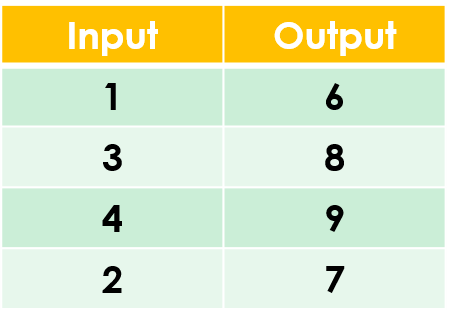1
visibility

Find the rule from the table below:• A

Add 8

• B

Add 5

The correct answer is "add 5".

Please Note:
Addition is the operation that increases a number by adding to it.

Solution:

To find the correct rule, we consider the given table.

We observe that the given rule makes each number increase. This implies addition.

We try adding 5 to each input number:

1 + 5 = 6
3 + 5 = 8
4 + 5 = 9
2 + 5 = 7

On adding 5 to the input numbers, we get the corresponding output numbers.

So, the rule is "add 5”.